# Olympiad Test: Elementary Mensuration-I -2

## 10 Questions MCQ Test Mathematics Olympiad Class 7 | Olympiad Test: Elementary Mensuration-I -2

Description
Attempt Olympiad Test: Elementary Mensuration-I -2 | 10 questions in 20 minutes | Mock test for Class 7 preparation | Free important questions MCQ to study Mathematics Olympiad Class 7 for Class 7 Exam | Download free PDF with solutions
QUESTION: 1

### What is the area of a right-angled triangle? I. The perimeter of the triangle is 30 cm. II. The ratio between the base and the height of the triangle is 5 : 12. III. The area of the triangle is equal to the area of a rectangle of length 10 cm.

Solution:

From II, base : height = 5 : 12
Let base = 5x and height = 12x
Then, hypotenuse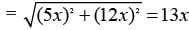From I, perimeter of the triangle = 30 cm.
∴ 5x + 12x + 13x = 30 ⇒ x = 1
So, base = 5x = 5 cm, height = 12x = 12 cm.
∴ Area = (1/2 × 5 × 12) = 30 cm2
Thus, I and II together give the answer.
Clearly III is redundant, since the breadth of the rectangle is not given.

QUESTION: 2

### What is the area of rectangular field? I. The perimeter of the field is 110 metres. II. The length is 5 metres more than the width. III. The ratio between length and width is 6 : 5 respectively.

Solution:

I. 2(l + b) = 110 l + b = 55
II. l = (b + 5) l – b = 5
III. l/b  = 6/5 5l – 6b = 0
These are three equations in l and b. We may solve them pair-wise.
∴  Any two of the three will give the answer.

QUESTION: 3

### What is the area of the given rectangle? I. Perimeter of the rectangle is 60 cm. II. Breadth of the rectangle is 12 cm. III. Sum of two adjacent sides is 30 cm.

Solution:

From I and II, we can find the length and breadth of the rectangle and therefore the area can be obtained.
So, III is redundant.
Also, from II and III, we can find the length and breadth and therefore the area can be obtained.
So, I is redundant.
∴ Correct answer is “II and either I or III”.

QUESTION: 4

What is the cost of painting the two adjacent walls of a hall at Rs 5 per m2, which has no windows or doors?
I. The area of the hall is 24 sq. m.
​II. The breadth, length and height of the hall are in the ratio of 4 : 6 : 5 respectively.
III. Area of one wall is 30 sq. m.

Solution:

From II, let l = 4x, b = 6x; and h = 5x.
Then, area of the hall = (24x2) m2.
From I. Area of the hall = 24 m2.
From II and I, we get 24x2 = 24 x = 1.
∴ l = 4 m, b = 6 and h = 5 m.
Thus, the area of two adjacent walls
= [(l × h) + (b × h)] m2 can be found out and so the cost of painting two adjacent walls may be found out.
Thus, III is redundant.

QUESTION: 5

Find the area of the largest circle that can be drawn in a square of side 14 cm.

Solution:

By the formula:
Required area = π (14/2)2 = 22/7 × 72
= 154 cm2

QUESTION: 6

In a quadrilateral, the length of one of its diagonal is 23 cm and the perpendiculars drawn on this diagonal from other two vertices measure 17 cm and 7 cm respectively. Find the area of the quadrilateral.

Solution:

Area of quadrilateral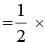any diagonal × (sum of perpendiculars drawn on diagonal from two vertices)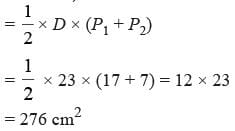QUESTION: 7

The circumference of a circle is 100 cm. Find the side of the square inscribed in the circle.

Solution:

Circumference of the circle = 2πr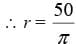∴ Side of the inscribed square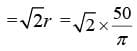QUESTION: 8

If the radius of a circle is increased by 5%, find the percentage increase in its area.

Solution:

% increase in its area
= 2 × 5 + 52/100
= 10 + 0.25 =10.25%

QUESTION: 9

If all sides of a hexagon is increased by 2%, find the percentage increase in its area.

Solution:

Required percentage increase
= 2 × 2 + 22/100
= 4 + 0.04
= 4.04%

QUESTION: 10

If diameter of a circle is increased by 12%, find the percentage increase in its circumference.

Solution:

Diameter is rarely used as the measuring side of a circle. Thus % increase in circumference =12%Use Code STAYHOME200 and get INR 200 additional OFF Use Coupon Code